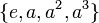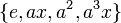# Simple fusion system for dihedral group:D8

(diff) ← Older revision | Latest revision (diff) | Newer revision → (diff)
This article describes a particular fusion system on a group of prime power order, namely dihedral group:D8.
Get information on fusion systems for dihedral group:D8.
View other particular fusion systems

This article describes a particular fusion system on dihedral group:D8 that turns out to be a simple fusion system: it has no normal fusion subsystems. This is the unique simple fusion system on the group.

## Explicit description

Equivalence class under isomorphisms, explicit description of subgroups Subgroups involved Order Index Number of conjugacy classes of subgroups fused Total number of subgroups (=1 iff weakly closed subgroup for the fusion system) Are all group automorphisms of each subgroup included? Size of automorphism group from the fusion system Total number of isomorphisms (including automorphisms and others) = (number of automorphisms)$\times$$\mbox{(number of subgroups)}^2$
trivial subgroup 1 8 1 1 Yes 1 1$\{ e, a^2 \}$$\{ e, x \}, \{ e, a^2x \}$$\{ e, ax \}, \{ e, a^3x \}$,
center of dihedral group:D8, non-normal subgroups of dihedral group:D8 (both conjugacy classes) 2 4 3 5 Yes 1 25$\{ e, a, a^2, a^3 \}$ cyclic maximal subgroup of dihedral group:D8 4 2 1 1 Yes 2 2$\{ e, x, a^2, a^2x \}$ Klein four-subgroups of dihedral group:D8 (one of them) 4 2 1 1 Yes 6 6$\{ e, ax, a^2, a^3x \}$ Klein four-subgroups of dihedral group:D8 (one of them) 4 2 1 1 Yes 6 6
whole group 8 1 1 1 No (see below) 4 4
Total -- -- 8 10 -- -- --

For the whole group, the only automorphisms that are included in the fusion system are the inner automorphisms. This can be traced to the fact that the outer automorphism group of the dihedral group is 2-group, and in particular has no 2'-automorphisms.

## Realization in groups

Group Order Dihedral group:D8 as a subgroup of this group Comment
projective special linear group:PSL(3,2) (same as PSL(2,7)) 168 D8 in PSL(3,2) example of smallest order, but not the only minimal example
alternating group:A6 360 D8 in A6 also a minimal example

## Element structure

### Fusion of elements

As far as elements are concerned, this fusion system is as rich as possible: any two elements of the same order get fused with each other. Below is some information. Each equivalence class under fusion is presented in terms of its conjugacy classes in the original group:

Equivalence class under fusion, i.e., equivalence class under conjugacy in the fusion system Order of elements Number of elements Number of conjugacy classes$\{ e \}$ 1 1 1$\{ a^2 \}$,$\{ x, a^2x \}$,$\{ ax, a^3x \}$ 2 5 3$\{ a, a^3 \}$ 4 2 1
Total -- 8 5